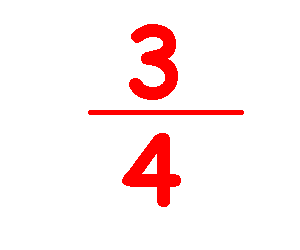*************************
Fractions - Part I

Fractions are not that difficult to understand.
They are written with a number on top (numerator), a line underneath, and a number on the bottom (denominator).
Here's a typical fraction:In this fraction, 3 is the numerator, 4 is the denominator, and it has the value of three fourths or 3 ÷ 4.

The easiest way to show how fractions work is with money.
In the United States and Canada, money is expressed in dollars and we can think of coins as fractions of a dollar.
A common coin is the quarter, so-called because it is worth a quarter of one dollar. (Look at a U.S. Quarter and you will see the words "quarter dollar").
From the Latin word "decimus" (meaning one tenth) we get the word dime which is one tenth of one dollar.
If we had 3 quarters, their total value would be 3/4 of one dollar. Three dimes would equal 3/10 of one dollar. If we wanted to find the total value of all 6 coins, we could easily add up 75¢ and 30¢ and find it equals \$1.05.

However, this is exactly the same as a math problem in which you have to find the sum of 3/4 and 3/10.
Notice that in each fraction, the bottom number denotes the type of coin it is, hence the term denominator. Also, you might have heard paper money (for example \$50 and \$100 bills) referred to as bills of large denominations.
Also notice that the top number in each fraction shows the amount, quantity or number of each coin we have, hence the term numerator. We could add the tops of the fractions and we would be correct in saying that it equals six coins but this does NOT give us the value.

So now we have to look for something that quarters and dimes have in common. We have to find a whole number into which we could convert quarters and dimes. The quarter does NOT work because a quarter equals 2 and a half dimes. However, nickels will work because a quarter equals 5 nickels and a dime equals 2 nickels. A nickel is one twentieth of a dollar (1/20) and so 3 dimes equal 6 nickels and three quarters have a value of 15 nickels. So, the nickel is the common denominator we had to find. We can show this mathematically as:
``` 3  +  3  =  15  +  6  =  21  = 1 plus  1
4    10     20    20     20           20

```
Yes, that was a long way to go just to add up six coins, but it does demonstrate how fractions work and now you know what the terms numerator, denominator and common denominator mean.

************************************

GO   TO   HOME   PAGE